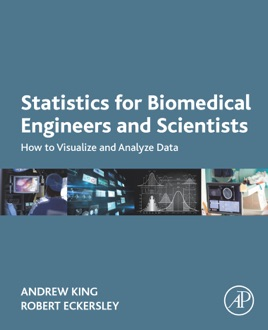• \$87.99

## Publisher Description

Statistics for Biomedical Engineers and Scientists: How to Analyze and Visualize Data provides an intuitive understanding of the concepts of basic statistics, with a focus on solving biomedical problems. Readers will learn how to understand the fundamental concepts of descriptive and inferential statistics, analyze data and choose an appropriate hypothesis test to answer a given question, compute numerical statistical measures and perform hypothesis tests ‘by hand’, and visualize data and perform statistical analysis using MATLAB. Practical activities and exercises are provided, making this an ideal resource for students in biomedical engineering and the biomedical sciences who are in a course on basic statistics.
Presents a practical guide on how to visualize and analyze statistical dataProvides numerous practical examples and exercises to illustrate the power of statistics in biomedical engineering applicationsGives an intuitive understanding of statistical testsCovers practical skills by showing how to perform operations ‘by hand’ and by using MATLAB as a computational toolIncludes an online resource with downloadable materials for students and teachers